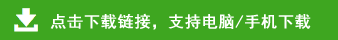# 小学二年级数学100道口算题

小学二年级数学100道口算题部分内容预览

42＋39=    53＋29=    92－37=    31－15=    27＋55=
19＋44=    25＋67=    46＋27=    85－26=    19＋34=
18＋66=    23－16=    93－25=    17＋69=    26＋45=
47＋15=    17＋79=    39＋26=    94－45=    37－19=
93－57=    37－29=    25＋57=    45＋17=    18＋46=
47＋25=    30－17=    27＋65=    85－59=    94－35=
84－49=    71－14=    46＋37=    71－24=    18＋56=
84－39=    69＋26=    93－47=    85－36=    53＋39=
27＋55=    19＋34=    26＋45=    37－19=    93－57=
18＋46=    94－35=    18＋56=    53＋39=    53＋29=
60－47=    75－37=    28＋27=    83－68=    61－34=
76－57=    82－48=    95－29=    35＋28=    29＋37=
44＋27=    75－47=    52－26=    19＋18=    27＋49=
52－36=    61－24=    96－17=    15＋68=    69＋23=
69＋13=    94－19=    37＋26=    67－48=    79＋19=
44＋37=    29＋14=    67－58=    83－58=    36＋16=
75－47＋57=   29＋37＋25=   60－47＋79=   93－55＋17=
93－55＋17=   94－58＋47=   86－59＋66=   81－32＋39=
47＋28＋18=   81－32＋39=   63＋28－68=   80－25－29=
73－14＋34=   29＋55－58=   63＋18－69=   91－75＋67=
63－38＋66=   80－25－29=   91－75＋67=   91－24＋24=